CAT  >  Test: CAT Logical Reasoning & Data Interpretation- 2

# Test: CAT Logical Reasoning & Data Interpretation- 2

Test Description

## 20 Questions MCQ Test CAT Mock Test Series | Test: CAT Logical Reasoning & Data Interpretation- 2

Test: CAT Logical Reasoning & Data Interpretation- 2 for CAT 2022 is part of CAT Mock Test Series preparation. The Test: CAT Logical Reasoning & Data Interpretation- 2 questions and answers have been prepared according to the CAT exam syllabus.The Test: CAT Logical Reasoning & Data Interpretation- 2 MCQs are made for CAT 2022 Exam. Find important definitions, questions, notes, meanings, examples, exercises, MCQs and online tests for Test: CAT Logical Reasoning & Data Interpretation- 2 below.
Solutions of Test: CAT Logical Reasoning & Data Interpretation- 2 questions in English are available as part of our CAT Mock Test Series for CAT & Test: CAT Logical Reasoning & Data Interpretation- 2 solutions in Hindi for CAT Mock Test Series course. Download more important topics, notes, lectures and mock test series for CAT Exam by signing up for free. Attempt Test: CAT Logical Reasoning & Data Interpretation- 2 | 20 questions in 40 minutes | Mock test for CAT preparation | Free important questions MCQ to study CAT Mock Test Series for CAT Exam | Download free PDF with solutions
 1 Crore+ students have signed up on EduRev. Have you?
Test: CAT Logical Reasoning & Data Interpretation- 2 - Question 1

### There are 7 oil wells which are connected as shown in the below diagram. The oil wells act only as storage and do not produce any oil by themselves. The whole distribution of oil starts from A as per the directions shown. Each of B, C, D, E and F oil wells have a limited capacity and the extra oil that flows into the oil well through the inflow pipes is distributed equally into all the outflow pipes. The maximum capacity of each oil pipe is 8 million litres. The maximum capacity of each oil well is marked in the diagram. The difference of the maximum capacity of a pipe and the oil that flows in that pipe is called the slack of that pipe. The pipes that are connected to A have an initial slack value of zero.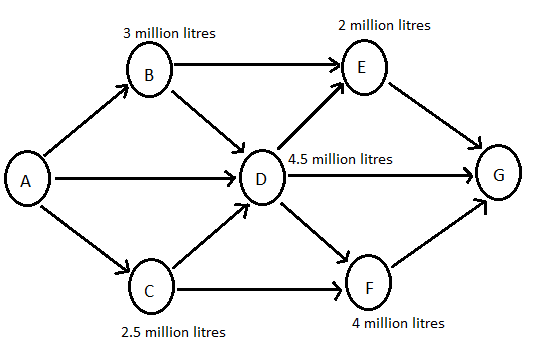Find the amount of oil that flow in the D-G pipeline.

Detailed Solution for Test: CAT Logical Reasoning & Data Interpretation- 2 - Question 1

We know that the slack in pipes A-B, A-C and A-D is zero. So, the volume of oil that flows in these three pipes is 8 million litres. B’s capacity is 3 million litres. So, the remaining 5 million litres is distributed into D and E equally. So, 2.5 million litres flow in both B-E and B-D pipes.
Similarly, the oil that flows into C is 8 million litres. C’s capacity is 2.5 million litres. So, the remaining 5.5 litres is distributed into D and F equally. So, 2.75 litres flow in both C-D and C-F pipes.
So, a total of 8 + 2.5 + 2.75 = 13.25 million litres has flown into D. D’s capacity is 4.5 million litres. The remaining 8.75 million litres is distributed into D-E, D-G and D-F pipes equally. So, 8.75/3 = 2.917 million litres flows in the D-G pipeline.

Test: CAT Logical Reasoning & Data Interpretation- 2 - Question 2

### There are 7 oil wells which are connected as shown in the below diagram. The oil wells act only as storage and do not produce any oil by themselves. The whole distribution of oil starts from A as per the directions shown. Each of B, C, D, E and F oil wells have a limited capacity and the extra oil that flows into the oil well through the inflow pipes is distributed equally into all the outflow pipes. The maximum capacity of each oil pipe is 8 million litres. The maximum capacity of each oil well is marked in the diagram. The difference of the maximum capacity of a pipe and the oil that flows in that pipe is called the slack of that pipe. The pipes that are connected to A have an initial slack value of zero.If the oil well G distributes all the oil that flows into it to 20 oil reservoirs, then what is the value of the oil that flows into each oil reservoir from G.

Detailed Solution for Test: CAT Logical Reasoning & Data Interpretation- 2 - Question 2

We know that the slack in pipes A-B, A-C and A-D is zero. So, the volume of oil that flows in these three pipes is 8 million litres. B’s capacity is 3 million litres. So, the remaining 5 million litres is distributed into D and E equally. So, 2.5 million litres flow in both B-E and B-D pipes.
Similarly, the oil that flows into C is 8 million litres. C’s capacity is 2.5 million litres. So, the remaining 5.5 litres is distributed into D and F equally. So, 2.75 litres flow in both C-D and C-F pipes.
So, a total of 8 + 2.5 + 2.75 = 13.25 million litres has flown into D. D’s capacity is 4.5 million litres. The remaining 8.75 million litres is distributed into D-E, D-G and D-F pipes equally. So, 8.75/3 = 2.917 million litres flows in the D-F pipeline.
So a total of 2.75 + 2.917 = 5.667 million litres flow into the F oil well. The capacity of F is 4 million litres. So, 5.667 - 4 = 1.667 million litres flow into the F-G pipeline.
The flow in D-E pipeline is 2.917 million litres and B-E pipeline is 2.5 million litres. So, a total of 5.417 million litres flows into E. The capacity of E is 2 million litres. So, the extra 3.417 million litres flows into E-G pipe.
So, a total of 3.417 + 2.917 + 1.667 = 8 million litres flows into G, which is distributed into 20 reservoirs. So, each reservoir has 8million/20 = 0.4 million litres.
Alternative approach:
The total flow into G cannot also be found by subtracting the requirement in each of the oil wells from the total outflow from A.
Total outflow from A = 3*8 = 24 million litres
Total requirement of B, C, D, E and F = 3 + 2.5 + 4.5 + 2 + 4 = 16 million litres
Total flow into G = 24 - 16 = 8 million litres, which is distributed into 20 reservoirs.
⇒ Each reservoir has 8 million/20 = 0.4 million litres.

Test: CAT Logical Reasoning & Data Interpretation- 2 - Question 3

### There are 7 oil wells which are connected as shown in the below diagram. The oil wells act only as storage and do not produce any oil by themselves. The whole distribution of oil starts from A as per the directions shown. Each of B, C, D, E and F oil wells have a limited capacity and the extra oil that flows into the oil well through the inflow pipes is distributed equally into all the outflow pipes. The maximum capacity of each oil pipe is 8 million litres. The maximum capacity of each oil well is marked in the diagram. The difference of the maximum capacity of a pipe and the oil that flows in that pipe is called the slack of that pipe. The pipes that are connected to A have an initial slack value of zero.The pipe C-D has a leakage problem and hence is closed. Find the slack in the D-G pipe in this situation.

Detailed Solution for Test: CAT Logical Reasoning & Data Interpretation- 2 - Question 3

We know that the slack in pipes A-B, A-C and A-D is zero. So, the volume of oil that flows in these three pipes is 8 million litres. B’s capacity is 3 million litres. So, the remaining 5 million litres is distributed into D and E equally. So, 2.5 million litres flow in both B-E and B-D pipes.
8 million litres come into D from A and 2.5 million litres come into D from B. Nothing comes from C because the C-D pipeline is closed. Hence the total volume into D is 10.5 million litres. The capacity of D is 4.5 million litres. So, 10.5 - 4.5 = 6 million litres is distributed into D-E, D-F and D-G pipelines equally. So, the flow in each of these pipelines is equal to 6/3 = 2 million litres. So, the slack in D-G pipeline is equal to 8 - 2 = 6 million litres.

*Answer can only contain numeric values
Test: CAT Logical Reasoning & Data Interpretation- 2 - Question 4

Instructions​ : Read the below information carefully and answer the following questions.
There are 100 students in a class. Each of the students has to opt for one or more of the three specializations among Finance, Operations and Marketing. The number of students who opted for Marketing is more than the number of students who opted for Finance which is more than the number of students who opted for Operations which is more than the number of students who opted for exactly two of the three specializations which is more than the number of students who opted for all three specializations. At least one student opted for all three specializations.
What is the maximum number of students who opted for Finance and Operations but not Marketing?

Detailed Solution for Test: CAT Logical Reasoning & Data Interpretation- 2 - Question 4

It is known that the total number of students is 100. We also know that the number of students who opted for Marketing is more than the number of students who opted for Finance which is more than the number of students who opted for Operations.
We have to maximize the number of students who chose both Finance and Operations as their specialization. We know that the number of students who chose Marketing will be greater than the number of students who opted for Finance and Operations as their specialization. This can be done as shown in the following Venn diagram.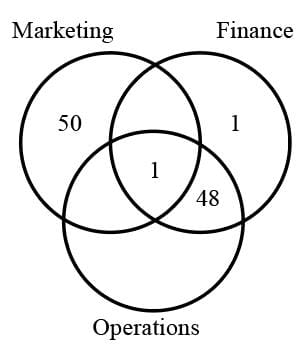Thus, the maximum number of students who opted for Finance and Operations but not Marketing = 48

*Answer can only contain numeric values
Test: CAT Logical Reasoning & Data Interpretation- 2 - Question 5

Instructions :
Consider 4 players A,B,C,D are playing a carrom match. The carrom match has 9 white, 9 black and 1 red coin. A,C are teammates and B,D are teammates. A round has four turns and a round is said to be completed when all the four players have completed their turns. The following is known about the rules of the carrom match.
a) A and C choose white colour for their team and A takes the first shot, followed by B,C,D respectively.
b) When a player pockets a coin of his chosen colour, he gets another chance in his turn.This continues until he does not pocket any coin, or when he pockets a coin of the opponents colour, which signals the end of his turn. The next player then starts his turn.
c) Any player who pockets the red coin, which is worth 5 points, should follow with pocketing a coin of his colour. If he pockets a coin of the opposite colour after pocketing the red, the red is awarded to the opposite team. If the player does not pocket any coin after pocketing the red, the red coin is placed back on to the board.
d) When there is only one coin of black or white and a red coin left on the board, the black/white coin should not be pocketed. Instead, the red coin is to be pocketed. If by mistake the lone coin is pocketed, it is to be placed back in the centre.
e) The game ends when one of the teams pockets all their coins. The points are calculated as follows.        No of points = (No of coins of opposite team left)*2 + points from pocketing red coin. The team with the most points wins.
Consider the case when 6 White coins have been pocketed at the end of the first round. (All players have had one turn each). What is the maximum number of coins that D could have pocketed in the first round, if the game does not end in the first round ?

Detailed Solution for Test: CAT Logical Reasoning & Data Interpretation- 2 - Question 5

We know that the game does not end in the first round, and at the end of the first round 3 white coins remain. This means that, for the game to not end, the minimum number of black coins that must be left on the board is 1.
Since D can pocket one white coin to end his turn, (A,B,C) in their turns pocket a total of 5 white coins and 0 black coins. This can be done using many combinations like (A,B,C) =  (3,0,2), (2,1,2) etc.
We now have to maximise the number of coins that D should pocket.
This means that D has to pocket all the black coins on the board except one, which has to be left on the board as the game does not end.
Therefore D should have pocketed 8 black coins, and also should also have pocketed the red coin during his turn.
Also, D should have ended his turn by pocketing a white coin at the end.
Therefore, the total number of coins that D could have pocketed is 8 black + 1 red + 1 white = 10 coins.

Test: CAT Logical Reasoning & Data Interpretation- 2 - Question 6

Instructions :
Consider 4 players A,B,C,D are playing a carrom match. The carrom match has 9 white, 9 black and 1 red coin. A,C are teammates and B,D are teammates. A round has four turns and a round is said to be completed when all the four players have completed their turns. The following is known about the rules of the carrom match.
a) A and C choose white colour for their team and A takes the first shot, followed by B,C,D respectively.
b) When a player pockets a coin of his chosen colour, he gets another chance in his turn.This continues until he does not pocket any coin, or when he pockets a coin of the opponents colour, which signals the end of his turn. The next player then starts his turn.
c) Any player who pockets the red coin, which is worth 5 points, should follow with pocketing a coin of his colour. If he pockets a coin of the opposite colour after pocketing the red, the red is awarded to the opposite team. If the player does not pocket any coin after pocketing the red, the red coin is placed back on to the board.
d) When there is only one coin of black or white and a red coin left on the board, the black/white coin should not be pocketed. Instead, the red coin is to be pocketed. If by mistake the lone coin is pocketed, it is to be placed back in the centre.
e) The game ends when one of the teams pockets all their coins. The points are calculated as follows.  No of points = (No of coins of opposite team left)*2 + points from pocketing red coin. The team with the most points wins.
Find the sum of all possible points that a team can score.

Detailed Solution for Test: CAT Logical Reasoning & Data Interpretation- 2 - Question 6

We consider all scores possible for Team A where the opponent is Team B.
Case 1: Red is pocketed by Team B
In such a case where the opponent has pocketed the red, then he must have taken at least one of his coins. Thus, the opposition team can have from 0 coins to 8 coins on board.
Therefore, all the possible scores of Team A are 0,2,4,6,......16.
Sum of this series = 2*(1+2+.. 8)= 2*36 = 72 points
Case 2: Red is pocketed by Team A
In this case, 0 to 9 coins of Team B can be on the board.
As the red is pocketed, then 5 points are added to each of the above scores. Hence, now, the possible scores are 5+2*0, 5+2*1 ... 5+2*9 = 5,7,9,......23 points. Sum of this series = 5*10 + 2*(1+2+...+9) = 50+90 = 140
The total is 72 + 140 = 212 points.

Test: CAT Logical Reasoning & Data Interpretation- 2 - Question 7

Instructions :
Consider 4 players A,B,C,D are playing a carrom match. The carrom match has 9 white, 9 black and 1 red coin. A,C are teammates and B,D are teammates. A round has four turns and a round is said to be completed when all the four players have completed their turns. The following is known about the rules of the carrom match.
a) A and C choose white colour for their team and A takes the first shot, followed by B,C,D respectively.
b) When a player pockets a coin of his chosen colour, he gets another chance in his turn.This continues until he does not pocket any coin, or when he pockets a coin of the opponents colour, which signals the end of his turn. The next player then starts his turn.
c) Any player who pockets the red coin, which is worth 5 points, should follow with pocketing a coin of his colour. If he pockets a coin of the opposite colour after pocketing the red, the red is awarded to the opposite team. If the player does not pocket any coin after pocketing the red, the red coin is placed back on to the board.
d) When there is only one coin of black or white and a red coin left on the board, the black/white coin should not be pocketed. Instead, the red coin is to be pocketed. If by mistake the lone coin is pocketed, it is to be placed back in the centre.
e) The game ends when one of the teams pockets all their coins. The points are calculated as follows.        No of points = (No of coins of opposite team left)*2 + points from pocketing red coin. The team with the most points wins.
If a white coin is the last coin to be pocketed in the game but Black wins and  k is the difference between the points of white team and black team, find the number of possible values for k?

Detailed Solution for Test: CAT Logical Reasoning & Data Interpretation- 2 - Question 7

If white coin is the last coin to be pocketed, this means that there are 0 white coins left on the board and at least one black coin on the board. In such a case, the points of black can be 5 or 0, depending on whether black has pocketed the red coin. As it is given that black wins, we can infer that the black team has pocketed the red coin. Hence, Black team points are 5.
Suppose there is 1 black coin left on the board, then, White's points would be 2.
Suppose there are 2 black coins left on the board, White would have 4 points. When 3 black coins exist , White would have 6 points. But this case would not exist as then White would win.
Hence, the point differences of Black - White can be 5-2 and 5-4.
The maximum number of values that can exist is 2.

Test: CAT Logical Reasoning & Data Interpretation- 2 - Question 8

There are 7 oil wells which are connected as shown in the below diagram. The oil wells act only as storage and do not produce any oil by themselves. The whole distribution of oil starts from A as per the directions shown. Each of B, C, D, E and F oil wells have a limited capacity and the extra oil that flows into the oil well through the inflow pipes is distributed equally into all the outflow pipes. The maximum capacity of each oil pipe is 8 million litres. The maximum capacity of each oil well is marked in the diagram. The difference of the maximum capacity of a pipe and the oil that flows in that pipe is called the slack of that pipe. The pipes that are connected to A have an initial slack value of zero.Find the slack in the F-G pipeline.

Detailed Solution for Test: CAT Logical Reasoning & Data Interpretation- 2 - Question 8

We know that the slack in pipes A-B, A-C and A-D is zero. So, the volume of oil that flows in these three pipes is 8 million litres. B’s capacity is 3 million litres. So, the remaining 5 million litres is distributed into D and E equally. So, 2.5 million litres flow in both B-E and B-D pipes.
Similarly, the oil that flows into C is 8 million litres. C’s capacity is 2.5 million litres. So, the remaining 5.5 litres is distributed into D and F equally. So, 2.75 litres flow in both C-D and C-F pipes.
So, a total of 8 + 2.5 + 2.75 = 13.25 million litres has flown into D. D’s capacity is 4.5 million litres. The remaining 8.75 million litres is distributed into D-E, D-G and D-F pipes equally. So, 8.75/3 = 2.917 million litres flows in the D-F pipeline.
So a total of 2.75 + 2.917 = 5.667 million litres flow into the F oil well. The capacity of F is 4 million litres. So, 5.667 - 4 = 1.667 million litres flow into the F-G pipeline. So, the slack of F-G pipeline = 8 - 1.667 = 6.333 million litres.

Test: CAT Logical Reasoning & Data Interpretation- 2 - Question 9

Instructions:
5 friends- Jack, Kiara, Liam, Mike and Nico went for a quiz competition and had to appear for an MCQ test, which was the first round for elimination. The test was fairly simple. It had just four questions and each question had 3 options- A, B and C. The five friends sat down in a continuous line, one behind the other, all facing the same direction. Except for the  person sitting in front among these 5 friends, all the others copied at least one answer from the person sitting immediately in front of him. Furthermore, for each of the pairs of friends sitting continuously, exactly one answer was same.
We are also given some additional facts-
1. Exactly two friends marked the answer as option B for Q1 and neither of the two were sitting at the first or the fifth position.
2.  The person sitting in the second position, who was not Liam, marked the answer as option B for Q3, which he copied.
3. Jack, who was sitting immediately in front of Liam, marked the answer as option C for exactly two questions, while Nico marked the answer as option C only for Q1.
4. The person sitting in the last position, who was not Liam, marked the answer as option B for Q2 and Mike, who was not in the last position, did not mark the answer to Q3 as option B.
5. Each of the four friends who copied the answer did so for a different question and each friend marked the answer as option A for at least one question, as option B for at least one question and as option C for at least one question.
6. The person sitting in the middle marked the answer to Q2 as option C, which he did not copy
7. The answers of two persons sitting one behind another are the same only if the person sitting at the back copied the answer.
What was the answer marked by the person sitting in the last position for Q4?

Detailed Solution for Test: CAT Logical Reasoning & Data Interpretation- 2 - Question 9

Let us use J, K, L, M and N as codes for Jack, Kiara, Liam, Mike and Nico respectively.
Using statements 2, 4 and 6, we can have the following table to start with:
Statement 2 gives that the person sitting in second position copied his third answer from the person before him. Hence person sitting in the first position must answer B for question 3.
Statement 6 mentions that the person sitting in the middle did not copy his answer.Since he answered C for question 2. The person sitting in front must not have answered C for question 2. Hence the person in position 2 for question can answer either A/B.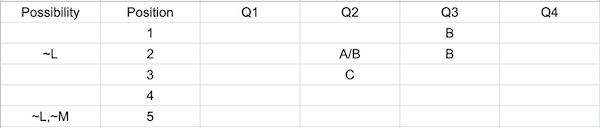Given J sits one place in front of L. Since L cannot sit at position 2 and 5 . J can sit only at 2,3. If J sits at 2 or 3 L must sit at 3 or 4.
Given in statement 4 M does not not sit position 5 nor answer option B for question 3. Hence he can only sit at either 3 or 4.
If J sits at 3 and L sits at 4 M does not have a place to sit. Hence J must sit at 2, L at 3 and M at 4.
Also, statement 3 says that J marked C twice and hence A and B were marked exactly once by him. Hence C must be answered for Q1 and Q4.
Statement 1 says that B was marked twice in question 1 hence it must be answered for by L and M because the person sitting at first and final position cannot answer B and person at position has already answered Q1 with C hence B must be answered for Q1 by persons L and M and since they are consecutively seated they must have copied. After placing as per the mentioned conditions the table looks like this :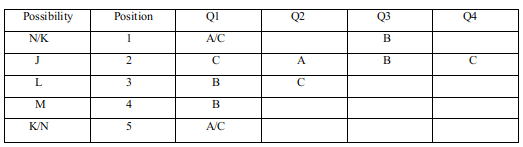Since the a set of persons must copy only one answer. The person sitting at position 1 cannot place C for Q1 because if he does that then there will be two copied answers between 1st and 2nd seated people. Hence he must answer A for question 1.
Note that in statement 3 it is mentioned that Nico marked the answer as option C only for Q1. Hence Nico is at position 5 and Kiara at position 1.
Also, exactly 2 persons marked B as answers to Q1. So,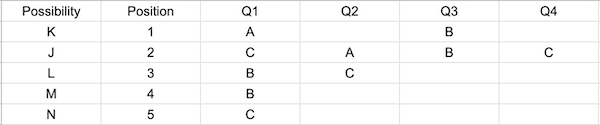Now, we have copied answers for pairs (3,4) and (1,2) and for questions 1 and 3.
So, pairs (2,3) and (4,5) must have copied answers for Q2 and Q4.
And since pair (2,3) did not copy answer for Q2, they must have done it for Q4. And the pair (4,5) must have copied for Q2.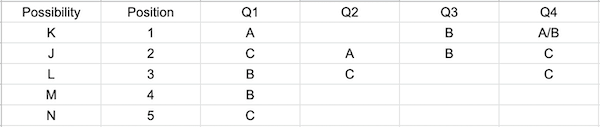Statement 4 tells that person sitting at the last position answers B for question 2. Since (4,5) copied Q2 person at position 4 must answer B for Q4
Now since, each of the 5 person must have at least one A, B and C each as the answers. We further deduce the following:
For M one of Q3 and Q4 must be answered with A and C. Since Q4 cannot be answered C by M as it would mean that he had copied this from L this is a false case. Hence he answers A for Q3 and C for Q4.
For L since we must have at least  one answer to be A we must Q3 with A.
For K we must have at least 1 answer to be C and we cannot answer Q4 with C we must answer Q2 with C.
The remaining Q4 for K can be answered with A/B but not C because J has answered Q4 with C.
The last person can answer Q3 with A/B because A/B because C cannot be placed as M answered Q3 with C similarly for Q4 only B/C can be used.
Also, the last person marked B as the answer for Q2. So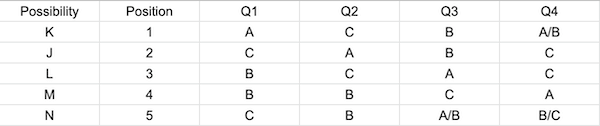Now, Nico only answered C for Q1. If he answers B for Q3 . He cannot answer A for any of the Questions hence he must answer Q3 with option A. Since he only answers one question with option C he must answer Q4 with B.
The final table looks like :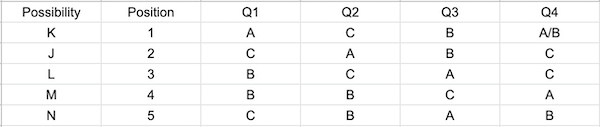Test: CAT Logical Reasoning & Data Interpretation- 2 - Question 10

Instructions:
5 friends- Jack, Kiara, Liam, Mike and Nico went for a quiz competition and had to appear for an MCQ test, which was the first round for elimination. The test was fairly simple. It had just four questions and each question had 3 options- A, B and C. The five friends sat down in a continuous line, one behind the other, all facing the same direction. Except for the  person sitting in front among these 5 friends, all the others copied at least one answer from the person sitting immediately in front of him. Furthermore, for each of the pairs of friends sitting continuously, exactly one answer was same.
We are also given some additional facts-
1. Exactly two friends marked the answer as option B for Q1 and neither of the two were sitting at the first or the fifth position.
2.  The person sitting in the second position, who was not Liam, marked the answer as option B for Q3, which he copied.
3. Jack, who was sitting immediately in front of Liam, marked the answer as option C for exactly two questions, while Nico marked the answer as option C only for Q1.
4. The person sitting in the last position, who was not Liam, marked the answer as option B for Q2 and Mike, who was not in the last position, did not mark the answer to Q3 as option B.
5. Each of the four friends who copied the answer did so for a different question and each friend marked the answer as option A for at least one question, as option B for at least one question and as option C for at least one question.
6. The person sitting in the middle marked the answer to Q2 as option C, which he did not copy
7. The answers of two persons sitting one behind another are the same only if the person sitting at the back copied the answer.
Which Question number did Kiara copy from her friend?

Detailed Solution for Test: CAT Logical Reasoning & Data Interpretation- 2 - Question 10

Let us use J, K, L, M and N as codes for Jack, Kiara, Liam, Mike and Nico respectively.
Using statements 2, 4 and 6, we can have the following table to start with:
Statement 2 gives that the person sitting in second position copied his third answer from the person before him. Hence person sitting in the first position must answer B for question 3.
Statement 6 mentions that the person sitting in the middle did not copy his answer.Since he answered C for question 2. The person sitting in front must not have answered C for question 2. Hence the person in position 2 for question can answer either A/B.Given J sits one place in front of L. Since L cannot sit at position 2 and 5 . J can sit only at 2,3. If J sits at 2 or 3 L must sit at 3 or 4.
Given in statement 4 M does not not sit position 5 nor answer option B for question 3. Hence he can only sit at either 3 or 4.
If J sits at 3 and L sits at 4 M does not have a place to sit. Hence J must sit at 2, L at 3 and M at 4.
Also, statement 3 says that J marked C twice and hence A and B were marked exactly once by him. Hence C must be answered for Q1 and Q4.
Statement 1 says that B was marked twice in question 1 hence it must be answered for by L and M because the person sitting at first and final position cannot answer B and person at position has already answered Q1 with C hence B must be answered for Q1 by persons L and M and since they are consecutively seated they must have copied. After placing as per the mentioned conditions the table looks like this :Since the a set of persons must copy only one answer. The person sitting at position 1 cannot place C for Q1 because if he does that then there will be two copied answers between 1st and 2nd seated people. Hence he must answer A for question 1.
Note that in statement 3 it is mentioned that Nico marked the answer as option C only for Q1. Hence Nico is at position 5 and Kiara at position 1.
Also, exactly 2 persons marked B as answers to Q1. So,Now, we have copied answers for pairs (3,4) and (1,2) and for questions 1 and 3.
So, pairs (2,3) and (4,5) must have copied answers for Q2 and Q4.
And since pair (2,3) did not copy answer for Q2, they must have done it for Q4. And the pair (4,5) must have copied for Q2.Statement 4 tells that person sitting at the last position answers B for question 2. Since (4,5) copied Q2 person at position 4 must answer B for Q4
Now since, each of the 5 person must have at least one A, B and C each as the answers. We further deduce the following:
For M one of Q3 and Q4 must be answered with A and C. Since Q4 cannot be answered C by M as it would mean that he had copied this from L this is a false case. Hence he answers A for Q3 and C for Q4.
For L since we must have at least one answer to be A we must Q3 with A.
For K we must have at least 1 answer to be C and we cannot answer Q4 with C we must answer Q2 with C.
The remaining Q4 for K can be answered with A/B but not C because J has answered Q4 with C.
The last person can answer Q3 with A/B because A/B because C cannot be placed as M answered Q3 with C similarly for Q4 only B/C can be used.
Also, the last person marked B as the answer for Q2. SoNow, Nico only answered C for Q1. If he answers B for Q3 . He cannot answer A for any of the Questions hence he must answer Q3 with option A. Since he only answers one question with option C he must answer Q4 with B.
The final table looks like :Since Kiara is the first person among the 5 friends, she did not copy any answer from her friends.

Test: CAT Logical Reasoning & Data Interpretation- 2 - Question 11

Instructions:
5 friends- Jack, Kiara, Liam, Mike and Nico went for a quiz competition and had to appear for an MCQ test, which was the first round for elimination. The test was fairly simple. It had just four questions and each question had 3 options- A, B and C. The five friends sat down in a continuous line, one behind the other, all facing the same direction. Except for the  person sitting in front among these 5 friends, all the others copied at least one answer from the person sitting immediately in front of him. Furthermore, for each of the pairs of friends sitting continuously, exactly one answer was same.
We are also given some additional facts-
1. Exactly two friends marked the answer as option B for Q1 and neither of the two were sitting at the first or the fifth position.
2.  The person sitting in the second position, who was not Liam, marked the answer as option B for Q3, which he copied.
3. Jack, who was sitting immediately in front of Liam, marked the answer as option C for exactly two questions, while Nico marked the answer as option C only for Q1.
4. The person sitting in the last position, who was not Liam, marked the answer as option B for Q2 and Mike, who was not in the last position, did not mark the answer to Q3 as option B.
5. Each of the four friends who copied the answer did so for a different question and each friend marked the answer as option A for at least one question, as option B for at least one question and as option C for at least one question.
6. The person sitting in the middle marked the answer to Q2 as option C, which he did not copy
7. The answers of two persons sitting one behind another are the same only if the person sitting at the back copied the answer.
For how many questions did Liam and the friend sitting in the first position mark the same answer?

Detailed Solution for Test: CAT Logical Reasoning & Data Interpretation- 2 - Question 11

Let us use J, K, L, M and N as codes for Jack, Kiara, Liam, Mike and Nico respectively.
Using statements 2, 4 and 6, we can have the following table to start with:
Statement 2 gives that the person sitting in second position copied his third answer from the person before him. Hence person sitting in the first position must answer B for question 3.
Statement 6 mentions that the person sitting in the middle did not copy his answer.Since he answered C for question 2. The person sitting in front must not have answered C for question 2. Hence the person in position 2 for question can answer either A/B.Given J sits one place in front of L. Since L cannot sit at position 2 and 5 . J can sit only at 2,3. If J sits at 2 or 3 L must sit at 3 or 4.
Given in statement 4 M does not not sit position 5 nor answer option B for question 3. Hence he can only sit at either 3 or 4.
If J sits at 3 and L sits at 4 M does not have a place to sit. Hence J must sit at 2, L at 3 and M at 4.
Also, statement 3 says that J marked C twice and hence A and B were marked exactly once by him. Hence C must be answered for Q1 and Q4.
Statement 1 says that B was marked twice in question 1 hence it must be answered for by L and M because the person sitting at first and final position cannot answer B and person at position has already answered Q1 with C hence B must be answered for Q1 by persons L and M and since they are consecutively seated they must have copied. After placing as per the mentioned conditions the table looks like this :Since the a set of persons must copy only one answer. The person sitting at position 1 cannot place C for Q1 because if he does that then there will be two copied answers between 1st and 2nd seated people. Hence he must answer A for question 1.
Note that in statement 3 it is mentioned that Nico marked the answer as option C only for Q1. Hence Nico is at position 5 and Kiara at position 1.
Also, exactly 2 persons marked B as answers to Q1. So,Now, we have copied answers for pairs (3,4) and (1,2) and for questions 1 and 3.
So, pairs (2,3) and (4,5) must have copied answers for Q2 and Q4.
And since pair (2,3) did not copy answer for Q2, they must have done it for Q4. And the pair (4,5) must have copied for Q2.Statement 4 tells that person sitting at the last position answers B for question 2. Since (4,5) copied Q2 person at position 4 must answer B for Q4
Now since, each of the 5 person must have at least one A, B and C each as the answers. We further deduce the following:
For M one of Q3 and Q4 must be answered with A and C. Since Q4 cannot be answered C by M as it would mean that he had copied this from L this is a false case. Hence he answers A for Q3 and C for Q4.
For L since we must have at least one answer to be A we must Q3 with A.
For K we must have at least 1 answer to be C and we cannot answer Q4 with C we must answer Q2 with C.
The remaining Q4 for K can be answered with A/B but not C because J has answered Q4 with C.
The last person can answer Q3 with A/B because A/B because C cannot be placed as M answered Q3 with C similarly for Q4 only B/C can be used.
Also, the last person marked B as the answer for Q2. SoNow, Nico only answered C for Q1. If he answers B for Q3 . He cannot answer A for any of the Questions hence he must answer Q3 with option A. Since he only answers one question with option C he must answer Q4 with B.
The final table looks like :Liam and Kiara has same answers for only Q2.

Test: CAT Logical Reasoning & Data Interpretation- 2 - Question 12

Instructions:
5 friends- Jack, Kiara, Liam, Mike and Nico went for a quiz competition and had to appear for an MCQ test, which was the first round for elimination. The test was fairly simple. It had just four questions and each question had 3 options- A, B and C. The five friends sat down in a continuous line, one behind the other, all facing the same direction. Except for the  person sitting in front among these 5 friends, all the others copied at least one answer from the person sitting immediately in front of him. Furthermore, for each of the pairs of friends sitting continuously, exactly one answer was same.
We are also given some additional facts-
1. Exactly two friends marked the answer as option B for Q1 and neither of the two were sitting at the first or the fifth position.
2.  The person sitting in the second position, who was not Liam, marked the answer as option B for Q3, which he copied.
3. Jack, who was sitting immediately in front of Liam, marked the answer as option C for exactly two questions, while Nico marked the answer as option C only for Q1.
4. The person sitting in the last position, who was not Liam, marked the answer as option B for Q2 and Mike, who was not in the last position, did not mark the answer to Q3 as option B.
5. Each of the four friends who copied the answer did so for a different question and each friend marked the answer as option A for at least one question, as option B for at least one question and as option C for at least one question.
6. The person sitting in the middle marked the answer to Q2 as option C, which he did not copy
7. The answers of two persons sitting one behind another are the same only if the person sitting at the back copied the answer.
For all the responses combined for the four questions, which option was the least chosen one among the three?

Detailed Solution for Test: CAT Logical Reasoning & Data Interpretation- 2 - Question 12

Let us use J, K, L, M and N as codes for Jack, Kiara, Liam, Mike and Nico respectively.
Using statements 2, 4 and 6, we can have the following table to start with:
Statement 2 gives that the person sitting in second position copied his third answer from the person before him. Hence person sitting in the first position must answer B for question 3.
Statement 6 mentions that the person sitting in the middle did not copy his answer.Since he answered C for question 2. The person sitting in front must not have answered C for question 2. Hence the person in position 2 for question can answer either A/B.Given J sits one place in front of L. Since L cannot sit at position 2 and 5 . J can sit only at 2,3. If J sits at 2 or 3 L must sit at 3 or 4.
Given in statement 4 M does not not sit position 5 nor answer option B for question 3. Hence he can only sit at either 3 or 4.
If J sits at 3 and L sits at 4 M does not have a place to sit. Hence J must sit at 2, L at 3 and M at 4.
Also, statement 3 says that J marked C twice and hence A and B were marked exactly once by him. Hence C must be answered for Q1 and Q4.
Statement 1 says that B was marked twice in question 1 hence it must be answered for by L and M because the person sitting at first and final position cannot answer B and person at position has already answered Q1 with C hence B must be answered for Q1 by persons L and M and since they are consecutively seated they must have copied. After placing as per the mentioned conditions the table looks like this :Since the a set of persons must copy only one answer. The person sitting at position 1 cannot place C for Q1 because if he does that then there will be two copied answers between 1st and 2nd seated people. Hence he must answer A for question 1.
Note that in statement 3 it is mentioned that Nico marked the answer as option C only for Q1. Hence Nico is at position 5 and Kiara at position 1.
Also, exactly 2 persons marked B as answers to Q1. So,Now, we have copied answers for pairs (3,4) and (1,2) and for questions 1 and 3.
So, pairs (2,3) and (4,5) must have copied answers for Q2 and Q4.
And since pair (2,3) did not copy answer for Q2, they must have done it for Q4. And the pair (4,5) must have copied for Q2.Statement 4 tells that person sitting at the last position answers B for question 2. Since (4,5) copied Q2 person at position 4 must answer B for Q4
Now since, each of the 5 person must have at least one A, B and C each as the answers. We further deduce the following:
For M one of Q3 and Q4 must be answered with A and C. Since Q4 cannot be answered C by M as it would mean that he had copied this from L this is a false case. Hence he answers A for Q3 and C for Q4.
For L since we must have at least one answer to be A we must Q3 with A.
For K we must have at least 1 answer to be C and we cannot answer Q4 with C we must answer Q2 with C.
The remaining Q4 for K can be answered with A/B but not C because J has answered Q4 with C.
The last person can answer Q3 with A/B because A/B because C cannot be placed as M answered Q3 with C similarly for Q4 only B/C can be used.
Also, the last person marked B as the answer for Q2. SoNow, Nico only answered C for Q1. If he answers B for Q3 . He cannot answer A for any of the Questions hence he must answer Q3 with option A. Since he only answers one question with option C he must answer Q4 with B.
The final table looks like :Option A was chosen 5/6 times.
Option B was chosen 8/7 times.
Option C was chosen 7 times.
So, even if Kiara marked A as the answer for Q4, A would still be the least chosen option.

Test: CAT Logical Reasoning & Data Interpretation- 2 - Question 13

Instructions:
Six friends- Aman, Billu, Dinesh, Ekansh, Ganesh and Hritesh went for a trip together across 5 different cities- Pune, Mumbai, Hyderabad, Delhi and Bangalore in that order. Each of the six friends made certain expenses in each of the five cities . It is known that the total amount remaining with each of the six friends before visiting Delhi was the same. The following graph shows the amount of money spent (in thousands ) in each city by the six friends: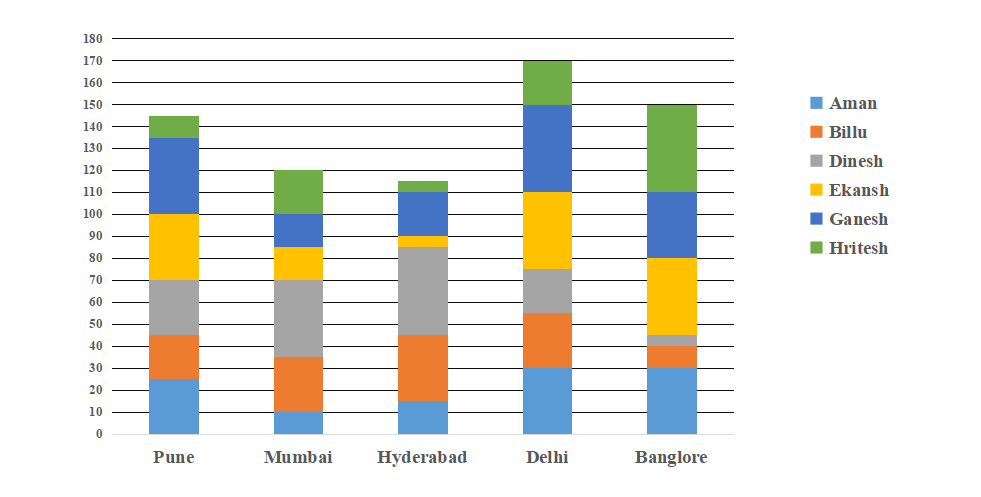Note : All values are either multiples of 5 or 10 (in thousands )
Which friend had the least amount with him before the start of the tour?

Detailed Solution for Test: CAT Logical Reasoning & Data Interpretation- 2 - Question 13

The amount of money (in thousands) spent by each of the friends in six cities as per the chart provided is: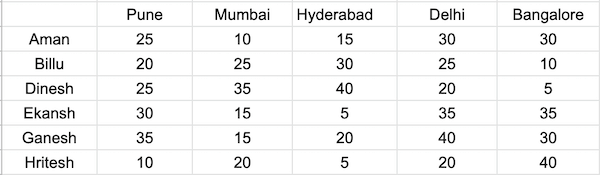After spending in Hyderabad, each of the six friends are left with same amount of money, say 'x'.
By then Aman, Billu, Dinesh, Ekansh, Ganesh and Hritesh have spent a total of 50, 75, 100, 50, 70 and 35 thousand respectively.
Since, they are left with the same amount after this, all of the six friends must have started the tour with (x+50), (x+75), (x+100), (x+50), (x+70) and (x+35) thousand respectively.
The following table shows the amount left with each of the friends after visiting the particular cities: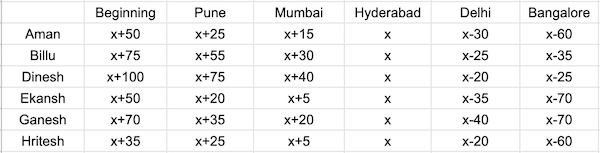Hritesh had the least amount before the start of the tour.

Test: CAT Logical Reasoning & Data Interpretation- 2 - Question 14

Instructions:
Six friends- Aman, Billu, Dinesh, Ekansh, Ganesh and Hritesh went for a trip together across 5 different cities- Pune, Mumbai, Hyderabad, Delhi and Bangalore in that order. Each of the six friends made certain expenses in each of the five cities . It is known that the total amount remaining with each of the six friends before visiting Delhi was the same. The following graph shows the amount of money spent (in thousands ) in each city by the six friends:Note : All values are either multiples of 5 or 10 (in thousands )
Which friend had the highest amount left with him after the tour to Bangalore?

Detailed Solution for Test: CAT Logical Reasoning & Data Interpretation- 2 - Question 14

The amount of money (in thousands) spent by each of the friends in six cities as per the chart provided is:After spending in Hyderabad, each of the six friends are left with same amount of money, say 'x'.
By then Aman, Billu, Dinesh, Ekansh, Ganesh and Hritesh have spent a total of 50, 75, 100, 50, 70 and 35 thousand respectively.
Since, they are left with the same amount after this, all of the six friends must have started the tour with (x+50), (x+75), (x+100), (x+50), (x+70) and (x+35) thousand respectively.
The following table shows the amount left with each of the friends after visiting the particular cities:After Bangalore's trip, Dinesh is left with the highest amount.

Test: CAT Logical Reasoning & Data Interpretation- 2 - Question 15

Instructions:
Six friends- Aman, Billu, Dinesh, Ekansh, Ganesh and Hritesh went for a trip together across 5 different cities- Pune, Mumbai, Hyderabad, Delhi and Bangalore in that order. Each of the six friends made certain expenses in each of the five cities . It is known that the total amount remaining with each of the six friends before visiting Delhi was the same. The following graph shows the amount of money spent (in thousands ) in each city by the six friends:Note : All values are either multiples of 5 or 10 (in thousands )
If each of the friends spent at least 50% of the amount (in all the trips including Bangalore) they initially had before the start of the tour,  what is the maximum amount of money with any friend before the beginning of Mumbai trip?

Detailed Solution for Test: CAT Logical Reasoning & Data Interpretation- 2 - Question 15

The amount of money (in thousands) spent by each of the friends in six cities as per the chart provided is: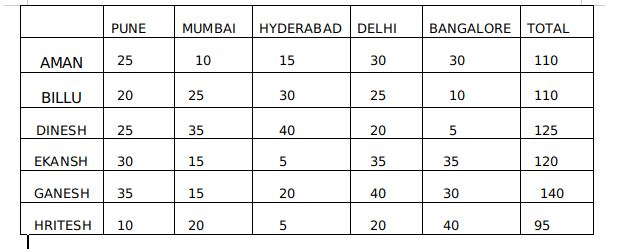Each spent at least 50% of money
Now Maximum amount will be left if each spent as less as possible which means each spent 50% of total money with him.
At the beginning of the trip they can have a maximum total of :
Aman  =220
Billu - 220
Dinesh -250
Ekansh- 240
Ganesh- 280
Hritesh - 190
But considering the additional condition that all of them have the same equal amounts by the time they reach Delhi :
Let initially their amounts be a, b, c, d, e, f
Since by the time they reach Delhi all of them must be equal :
By the time they reach Delhi
For Aman : a - 50000
If Aman has 220000 he will end up with 220000- 50000 = 170000 by the time he reaches Delhi. This is the maximum limit.
For Billu : b - 75000
If Billu has 220000 he will end up with 220000- 75000 = 145000 by the time he reaches Delhi. This is the maximum limit.
For Dinesh : c - 100000
If Dinesh has 250000 he will end up with 250000- 100000 = 150000 by the time he reaches Delhi. This is the maximum limit.
For Ekansh : d - 50000
If Ekansh has 240000 he will end up with 240000- 50000 = 190000 by the time he reaches Delhi. This is the maximum limit.
For Ganesh : e - 70000

If Ganesh has 280000 he will end up with 280000- 70000 = 210000 by the time he reaches Delhi. This is the maximum limit.
For Hritesh : f - 35000
If Hritesh has 190000 he will end up with 190000- 35000 = 155000 by the time he reaches Delhi. This is the maximum limit.
The lowest among the maximum for all the people was for Billu which was 145000.
Hence everyone must have Rs 145000 by the time they reach Delhi.
Before the beginning of Mumbai trip :
Aman will have 145000 + 10000 + 15000 =  Rs 170000
Billu will have 145000 + 30000 + 25000 = Rs 200000
Dinesh will have 145000 + 40000 + 35000 = Rs 220000
Ekansh will have 145000 + 15000 + 5000 = Rs 165000
Ganesh will have 145000 + 15000 + 20000 = Rs 180000
Hritesh will have 145000 + 20000 + 5000 = Rs 170000
Among these Dinesh has the highest of Rs 220000 before the Mumbai trip.

Test: CAT Logical Reasoning & Data Interpretation- 2 - Question 16

Instructions:
Six friends- Aman, Billu, Dinesh, Ekansh, Ganesh and Hritesh went for a trip together across 5 different cities- Pune, Mumbai, Hyderabad, Delhi and Bangalore in that order. Each of the six friends made certain expenses in each of the five cities . It is known that the total amount remaining with each of the six friends before visiting Delhi was the same. The following graph shows the amount of money spent (in thousands ) in each city by the six friends:Note : All values are either multiples of 5 or 10 (in thousands )
If Ganesh was left with Rs. 30,000 at the end of the entire trip, what is the amount of money left with Billu after his trip to Mumbai?

Detailed Solution for Test: CAT Logical Reasoning & Data Interpretation- 2 - Question 16

The amount of money (in thousands) spent by each of the friends in six cities as per the chart provided is:After spending in Hyderabad, each of the six friends are left with same amount of money, say 'x'.
By then Aman, Billu, Dinesh, Ekansh, Ganesh and Hritesh have spent a total of 50, 75, 100, 50, 70 and 35 thousand respectively.
Since, they are left with the same amount after this, all of the six friends must have started the tour with (x+50), (x+75), (x+100), (x+50), (x+70) and (x+35) thousand respectively.
The following table shows the amount left with each of the friends after visiting the particular cities:Ganesh is left with Rs. (x-70) thousand after the trip ends.
So, x=Rs. 100,000
Amount left with Billu after his trip to Mumbai= Rs. (x+30) thousand= Rs. 130,000

Test: CAT Logical Reasoning & Data Interpretation- 2 - Question 17

Refer to the data below and answer the questions that follow.
Rajesh has a cube having length of each side equal to 5 cm. Out of the three pairs of opposite faces, one is painted Red, other is painted Blue while the third is painted Green.
Rajesh placed the cube such that one green face of the cube was touching the ground and one red face of the cube was directly in front of him. He further cut the cube into 125 small identical cubes having each side equal to 1 cm. He then assigned numbers to the 125 small cubes as follows:
The cubes in the front-most row of the bottommost layer were assigned numbers 1 to 5 from left to right. The cubes in the row just behind the front-most row of the bottommost layer were assigned numbers 6 to 10 from left to right. The cubes in the middle row of the bottommost layer were assigned numbers 11 to 15 from left to right. The numbering was continued this way and the rightmost cube in the last row of the bottommost layer was assigned number 25.
Assignment of the numbers was continued for the second layer from the bottom on similar lines. The leftmost cube in the front-most row of the second layer from the bottom was assigned 26, the rightmost cube in the front-most row of the second layer from the bottom was assigned 30 while the rightmost cube in the last row of the second layer from the bottom was assigned 50.
The numbering was continued on similar lines for the remaining layers of the cubes. The leftmost cube in the front-most row of the topmost layer of the cubes was assigned 101 while the rightmost cube in the last row of the topmost layer was assigned 125.

What is the sum of the numbers assigned to the cubes that have green colour on at least one of their faces?

Detailed Solution for Test: CAT Logical Reasoning & Data Interpretation- 2 - Question 17

The bottom and top faces of the cube have been painted using green colour. The numbers assigned to the cubes on the bottom face are 1 to 25 while the numbers assigned to the cubes on the top face are 101 to 125.
Therefore, the required sum = 25 x 26/2  + 101 + 125/2  x  25 = 3150
Hence, .
Alternatively,
Each tube of the top face is numbered 100 more the cube which is in its position at the bottom face. If sum of cubes of bottom face is x, sum of cubes of the top face = x + 25 × 100.
The required sum is more than 2500. 3150 is the only option greater than 2500.

*Answer can only contain numeric values
Test: CAT Logical Reasoning & Data Interpretation- 2 - Question 18

The number of ways in which 16 sovereigns can be distributed among 3 applicants such that each applicant does not receive less than 3 sovereigns is:

Detailed Solution for Test: CAT Logical Reasoning & Data Interpretation- 2 - Question 18

Let x, y, z be the number of sovereigns received by the 3 applicants. Then x≥3,y≥3,z≥3 and x+y+z=16.
Let u=x-3,v=y-3and w=z-3,then u≥0,v≥0,w≥0
So, u+3+v+3+w+3=16
Or, u+v+w=7
The total number of the solutions of the given equation is 7+3-1C3-1 = 9C2 = 36.

*Answer can only contain numeric values
Test: CAT Logical Reasoning & Data Interpretation- 2 - Question 19

An amount triples in 4 years under compound interest at a certain rate of interest, the interest is being compounded annually, the time it would take to become 9 times itself.

Detailed Solution for Test: CAT Logical Reasoning & Data Interpretation- 2 - Question 19

It is given that the sum triples in 4 years. If it has become 9 times itself, it was tripled twice. Let the amount be P, and it gets tripled in 4 years at R% p.a.
So P(1 + (r/100))4 = 3P
Or, (1 + (R/100))4 = 3
Let it take n years to become 9 times.
Or, P(1 + (R/100))n = 9P
Or, (1 + R/100)n = 9
Or, [(1 + (R/100))4]n/4 = 32
Or, 3n/4 = 32 or, n/4 = 2
Or n = 8.

*Answer can only contain numeric values
Test: CAT Logical Reasoning & Data Interpretation- 2 - Question 20

The faces of a cube of n cm is first painted red and then the cube is cut into smaller cubes of 1cm. If the difference between the number of cubes with 1 face painted and the number with 2 faces painted is 90, what is the number of cubes with no face painted?

Detailed Solution for Test: CAT Logical Reasoning & Data Interpretation- 2 - Question 20

For n cm sized cube the number of cubes with 3 faces painted = 8
Number of cubes with 2 faces painted = 12x(n-2)
Number of cubes with 1 face painted = 6(n-2)2
Number of cubes with 0 face painted = (n-2)3
Given 6(n-2)2 - 12(n-2) = 90
So (n-2)(n-4) = 15
Or n = 7
Therefore number of cubes with 0 face painted = (7-2)3 = 125

## CAT Mock Test Series

2 videos|24 docs|89 tests
 Use Code STAYHOME200 and get INR 200 additional OFF Use Coupon Code
Information about Test: CAT Logical Reasoning & Data Interpretation- 2 Page
In this test you can find the Exam questions for Test: CAT Logical Reasoning & Data Interpretation- 2 solved & explained in the simplest way possible. Besides giving Questions and answers for Test: CAT Logical Reasoning & Data Interpretation- 2, EduRev gives you an ample number of Online tests for practice

## CAT Mock Test Series

2 videos|24 docs|89 tests

### How to Prepare for CAT

Read our guide to prepare for CAT which is created by Toppers & the best Teachers